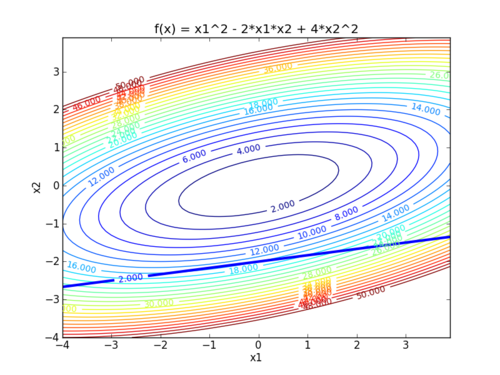# Main: Karush-Kuhn-Tucker (KKT) Conditions

The necessary conditions for a constrained local optimum are called the Karush Kuhn Tucker (KKT) Conditions, and these conditions play a very important role in constrained optimization theory and algorithm development. For an optimization problem:

$$\min_x f(x)$$ $$\mathrm{subject\;to}\quad g_i(x)-b_i \ge 0 \quad i=1,\ldots,k$$ $$\quad\quad\quad\quad\quad g_i(x)-b_i = 0 \quad i=k+1,\ldots,m$$

There are four KKT conditions for optimal primal (x) and dual (\lambda) variables. The asterisk (*) denotes optimal values.

##### 1. Feasible Constraints

$$g_i(x^*)-b_i \mathrm{\;is\;feasible}$$

##### 2. No Feasible Descent

$$\nabla f(x^*)-\sum_{i=1}^m \lambda_i^* \nabla g_i\left(x^*\right)=0$$

##### 3. Complementary Slackness

$$\lambda_i^* \left( g_i(x^*)-b_i \right) = 0$$

##### 4. Positive Lagrange Multipliers

$$\lambda_i^* \ge 0$$

The feasibility condition (1) applies to both equality and inequality constraints and is simply a statement that the constraints must not be violated at optimal conditions. The gradient condition (2) ensures that there is no feasible direction that could potentially improve the objective function. The last two conditions (3 and 4) are only required with inequality constraints and enforce a positive Lagrange multiplier when the constraint is active (=0) and a zero Lagrange multiplier when the constraint is inactive (>0).#### Part 1: 5 Minute Tutorial on the KKT Conditions

This 5 minute introductory video reviews the 4 KKT conditions and applies them to solve a simple quadratic programming (QP) problem with:

• 2 Linear equality constraints
• 3 Variables (x1, x2, x3)

Download the following worksheet on KKT conditions. The video below reviews the solution to this worksheet.

#### Part 2: 5 Minute Tutorial with KKT Conditions and Inequality Constraints

This next 5 minute introductory is similar to the previous one but solves a problem with inequality constraints instead of equality constraints. The problem is a simple quadratic programming (QP) problem with:

• 2 Linear inequality constraints
• 3 Variables (x1, x2, x3)

Download the following worksheet on KKT conditions with inequality constraints. The video below reviews the solution to this worksheet.

#### Part 3: 5 Minute KKT Exercise with both Inequality and Equality Constraints

This 5 minute exercise is similar to the previous ones but solves a problem with both equality and inequality constraints.

Download the following worksheet on KKT conditions with inequality and equality constraints. The video below reviews the solution to this worksheet.

#### Part 4: 5 Minute Application Exercise for the Optimal Volume of a Tank

This 5 minute exercise covers an application to a tank volume optimization. In this case, we specify the final Lagrange multiplier of \$8/ft3.

Download the following worksheet on this application of the KKT conditions. The video below reviews the solution to this worksheet.

#### Part 5: KKT Conditions for Dynamic OptimizationThis assignment can be completed in groups of two. Additional guidelines on individual, collaborative, and group assignments are provided under the Expectations link.